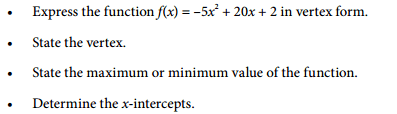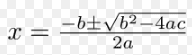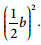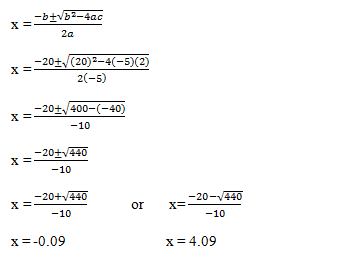# Function: expressing functions in vertex form.

• Evangeline101

## Homework Statement2. Homework Equations## The Attempt at a Solution

a) [/B]f(x) = -5x2 + 20x + 2

y = -5x2 + 20x + 2

Factor -5 from the first two terms:

y = -5x2 + 20x + 2

= -5 (x2 – 4x) +2

Complete the square in the bracket:

(1/2 b)2 = [1/2 (-4)]2 = (-2)2 = 4

Group the perfect square trinomial:

= -5 (x2 – 4x + 4 – 4) +2

Remove -4 from the brackets, it must be multiplied by -5:

= -5 [(x2 – 4x + 4) – 4] +2

= - 5 (x2 – 4x + 4) + 20 + 2

Factor the trinomial. Add remaining constants:

= -5 (x-2)2 + 22

b) The vertex is (2, 22)

c) Since the parabola is concave down, the maximum value is y = 22

d)f(x) = -5x2 + 20x + 2

y = -5x2 + 20x + 2

Let y = 0

0 = -5x2 + 20x + 2

Substitute a = -5, b = 20, c = 2 into the quadratic formula:The x-intercepts are x= -0.09 and x = 4.09

Is this correct?

Thanks.

#### Attachments

Correct. You really should check by graphing instead of relying on others.

You could also verify the x-intercept values by subbing them into the original equation. The whole thing should be equivalent to 0.